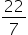Maths-
General
Easy

Question

# There are two circle with same centre O. The radius of larger circle is 4cm and that of the smaller circle is 2cm.Find area of the shaded region.## The correct answer is: 37.7 sq cm

### ar. of shaded region = ar. of larger circle – ar. of smaller circle{ar. of circle =× r × r}=× 4 × 4 -× 2 × 2=(16 - 4)=× 12= 37.7 sq. cm#### With Turito Foundation.#### Get an Expert Advice From Turito.